1:41 AM
Parametric Resonance

# Parametric Resonance

Michael Fowler

## Introduction

(Following Landau para 27)

A one-dimensional simple harmonic oscillator, a mass on a spring,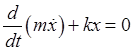has two parameters,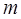andFor some systems, the parameters can be changed externally (an example being the length of a pendulum if at the top end the string goes over a pulley).

We are interested here in the system's response to some externally imposed periodic variation of its parameters, and in particular we'll be looking at resonant response, meaning large response to a small imposed variation.

Note first that imposed variation in the mass term is easily dealt with, by simply redefining the time variable to, meaning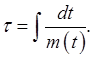Then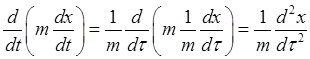,

and the equation of motion becomes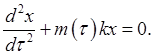This means we can always transform the equation so all the parametric variation is in the spring constant, so we'll just analyze the equationFurthermore, since we're looking for resonance phenomena, we will only consider a small parametric variation at a single frequency, that is, we'll takewhere, and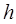is positive (a trivial requirement -- just setting the time origin).

(Note: We preferwhere Landau useswhich is often used for a resonance width these days.)

We have now a driven oscillator: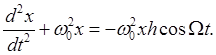How does this differ from our previous analysis of a driven oscillator? In a very important way!

The amplitude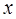is a factor in the driving force.

For one thing, this means that if the oscillator is initially at rest, it stays that way, in contrast to an ordinary externally driven oscillator. But if the amplitude increases, so does the driving force. This can lead to an exponential increase in amplitude, unlike the linear increase we found earlier with an external driver. (Of course, in a real system, friction and nonlinear potential terms will limit the growth.)

What frequencies will prove important in driving the oscillator to large amplitude? It responds best, of course, to its natural frequency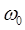. But if it is in fact already oscillating at that frequency, then the driving force, including the factor of, is proportional to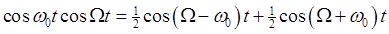,

with no component at the natural frequencyfor a general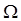.

The simplest way to get resonance is to takeCan we understand this physically? Yes. Imagine a mass oscillating backwards and forwards on a spring, and the spring force increases just after those points where the mass is furthest away from equilibrium, so it gets an extra tug inwards twice a cycle. This will feed in energy. (You can drive a swing this way.) In contrast, if you drive at the natural frequency, giving little push inwards just after it begins to swing inwards from one side, then you'll be giving it a little push outwards just after it begins to swing back from the other side. Of course, if you push only from one side, like swinging a swing, this works -- but it isn't a single frequency force, the next harmonic is doing most of the work.

## Resonance near Double the Natural Frequency

From the above argument, the place to look for resonance is close toLandau takes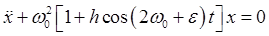and, bearing in mind that we're looking for oscillations close to the natural frequency, puts in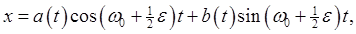with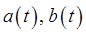slowly varying.

It's important to realize that this is an approximate approach. It neglects nonresonant frequencies which must be present in small amounts, for example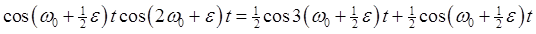and the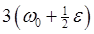term is thrown away.

And, since the assumption is thatare slowly varying, their second derivatives are dropped too, leaving justThis must equal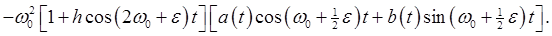Keeping only the resonant terms, we take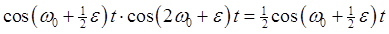andso this expression becomesThe equation becomes:The zeroth-order terms cancel between the two sides, leaving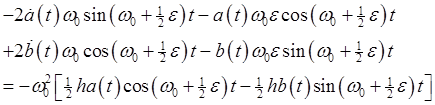Collecting the terms in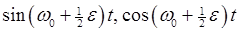:The sine and cosine can't cancel each other, so the two coefficients must both be identically zero. This gives two first order differential equations for the functions, and we look for exponentially increasing functions, proportional to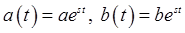, which will be solutions provided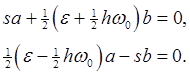The amplitude growth rate is thereforeParametric resonance will take place if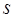is real, that is, if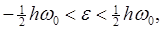a band of widthabout.

## Example: Pendulum Driven at near Double the Natural Frequency

A simple pendulum of length, massis attached to a point which oscillates vertically. Measuringdownwards, the pendulum position is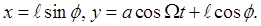The LagrangianThe purely time-dependent term will not affect the equations of motion, so we drop it, and since the equations are not affected by adding a total derivative to the Lagrangian, we can integrate the second term by parts (meaning we're dropping a term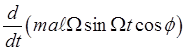) to get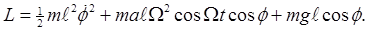(We've also dropped the termfrom the potential energy term -- it has noor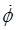dependence, so will not affect the equations of motion.)

The equation for small oscillations isComparing this withwe see that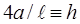, so the parametric resonance range aroundis of widthCategory: Education | Views: 347 | Added by: farrel | | Rating: 0.0/0